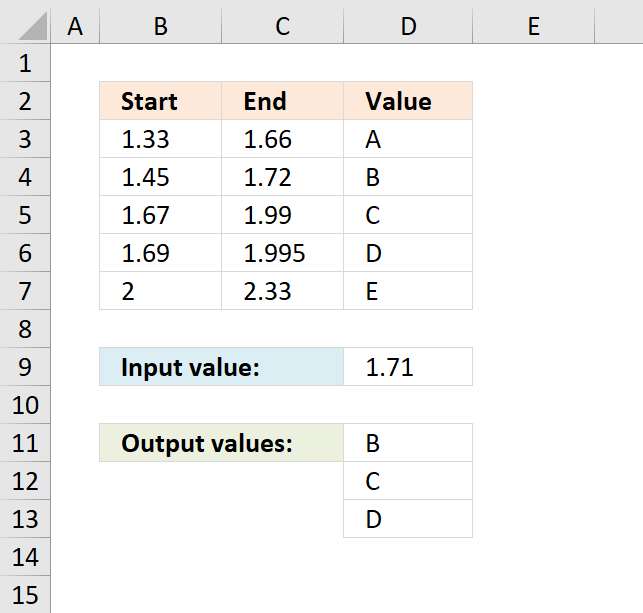Author: Oscar Cronquist Article last updated on December 12, 2018The image above shows a formula in cell C11 that extracts values from column D if the number in cell D9 is in a range specified in B3:B7 and C3:C7. This formula extracts multiple values if multiple ranges match.

The example above extracts B, C and D because 1.71 is between 1.45 - 1.72 (B), 1.67-1.99 (C) and 1.69-1.995 (D).

Array formula in cell C11:

=INDEX(\$D\$3:\$D\$7, SMALL(IF((\$B\$3:\$B\$7<=\$D\$9)*(\$C\$3:\$C\$7>=\$D\$9), MATCH(ROW(\$D\$3:\$D\$7), ROW(\$D\$3:\$D\$7)), ""), ROWS(\$A\$1:A1)))

### Explaining formula in cell C11

#### Step 1 - Check if number is larger than or equal to numbers in column B

The less than sign and the equal sign compares the numbers, if a number in column B is smaller than or equal to number in cell D9 then it evaluates to TRUE, if not FALSE.

\$B\$3:\$B\$7<=\$D\$9

becomes

{1.33;1.45;1.67;1.69;2}<=1.71

and returns

{TRUE; TRUE; TRUE; TRUE; FALSE}.

#### Step 2 - Check if number is smaller than or equal to numbers in column C

\$C\$3:\$C\$7>=\$D\$9

becomes

{1.66;1.72;1.99;1.995;2.33}>=1.71

and returns

{FALSE; TRUE; TRUE; TRUE; TRUE}

#### Step 3 - AND logic

Now we multiply the arrays, both logical expression must evaluate to TRUE in order to get the correct value(s). The parentheses are there to make sure that the correct calculation order is maintained.

(\$B\$3:\$B\$7<=\$D\$9)*(\$C\$3:\$C\$7>=\$D\$9)

becomes

{TRUE; TRUE; TRUE; TRUE; FALSE}*{FALSE; TRUE; TRUE; TRUE; TRUE}

and returns {0;1;1;1;0}.

#### Step 4 - Replace TRUE with row number

The IF function returns a unique number if boolean value is TRUE. FALSE returns "" (nothing).

IF((\$B\$3:\$B\$7<=\$D\$9)*(\$C\$3:\$C\$7>=\$D\$9), MATCH(ROW(\$D\$3:\$D\$7), ROW(\$D\$3:\$D\$7)), "")

becomes

IF({0;1;1;1;0}, MATCH(ROW(\$D\$3:\$D\$7), ROW(\$D\$3:\$D\$7)), "")

becomes

IF({0;1;1;1;0}, {1;2;3;4;5}, "")

and returns {"";2;3;4;""}.

#### Step 5 - Extract k-th smallest row number

The SMALL function lets you calculate the k-th smallest value from a cell range or array. SMALL( arrayk)

SMALL(IF((\$B\$3:\$B\$7<=\$D\$9)*(\$C\$3:\$C\$7>=\$D\$9), MATCH(ROW(\$D\$3:\$D\$7), ROW(\$D\$3:\$D\$7)), ""), ROWS(\$A\$1:A1))

becomes

SMALL({"";2;3;4;""},  ROWS(\$A\$1:A1))

The ROWS function keeps track of the numbers based on an expanding cell reference. It will expand as the formula is copied to the cells below.

SMALL({"";2;3;4;""},  ROWS(\$A\$1:A1))

becomes

SMALL({"";2;3;4;""},  1)

and returns 2.

#### Step 6 - Get corresponding value

The INDEX function returns a value based on row number (and column number if needed)

INDEX(\$D\$3:\$D\$7, SMALL(IF((\$B\$3:\$B\$7<=\$D\$9)*(\$C\$3:\$C\$7>=\$D\$9), MATCH(ROW(\$D\$3:\$D\$7), ROW(\$D\$3:\$D\$7)), ""), ROWS(\$A\$1:A1)))

becomes

INDEX(\$D\$3:\$D\$7, 2)

and returns "B" in cell C11.

### Download Excel *.xlsx file

Return multiple values if in range.xlsx